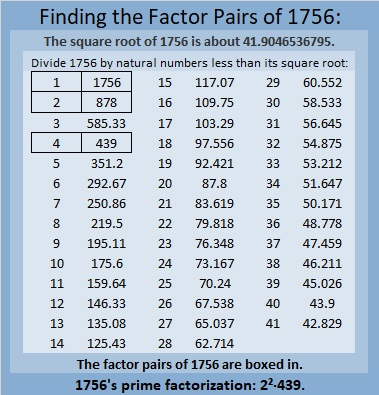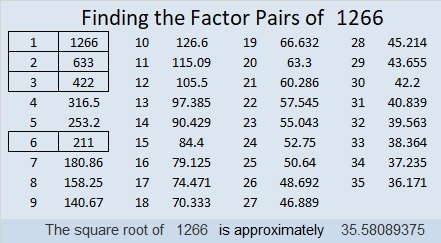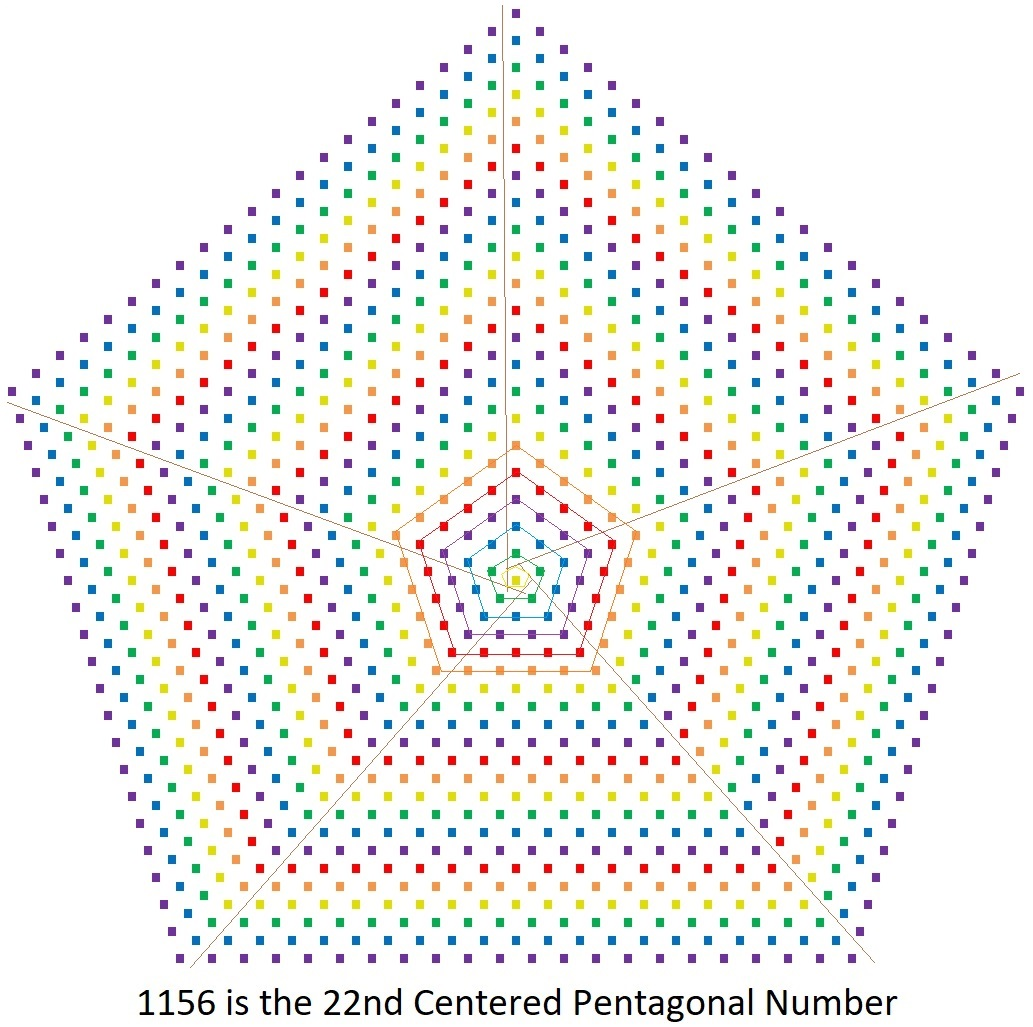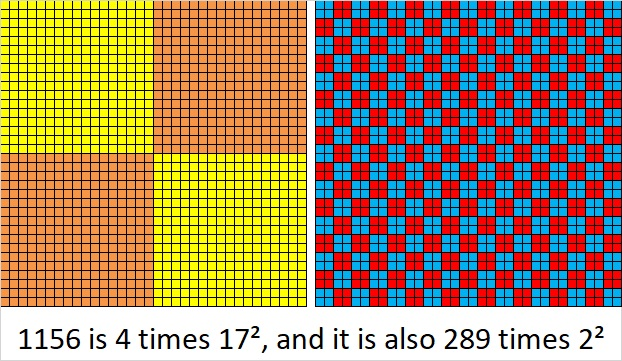# When Does 1756 Look a Little Like π?

### Today’s Puzzle:

Here are 1756 tiny squares. Solve for n and you will know which centered pentagonal number 1756 is. You could also count the pentagons from the center outward.What triangular number multiplied by 5 is one less than 1756?

How do you pronounce pentagonal? Here’s a quick video on its correct pronunciation:

Confession: I had been mispronouncing all of those terms, but not anymore!

### Factors of 1756:

• 1756 is a composite number.
• Prime factorization: 1756 = 2 × 2 × 439, which can be written 1756 = 2² × 439.
• 1756 has at least one exponent greater than 1 in its prime factorization so √1756 can be simplified. Taking the factor pair from the factor pair table below with the largest square number factor, we get √1756 = (√4)(√439) = 2√439.
• The exponents in the prime factorization are 2 and 1. Adding one to each exponent and multiplying we get (2 + 1)(1 + 1) = 3 × 2 = 6. Therefore 1756 has exactly 6 factors.
• The factors of 1756 are outlined with their factor pair partners in the graphic below.### More About the Number 1756:

The centered pentagon above doesn’t really look like π, so when does 1756 look a little bit like π? Pi day in America is next week, so this is a time-sensitive question.

Actually,1756 looks a little like 10π in base 24. You see 1756₁₀ is the same as 314₂₄.
Why? Because 3(24²) + 1(24) + 4(1) = 1756.

1756₁₀ also is the same as 1024₁₂.
Why? Because 1(12³) + 0(12²) + 2(12) + 4(1) = 1756.
I think that’s cool because 2¹º = 1024.

1756 is the difference of two squares:
440² – 438² = 1456.

# 1266 is a Centered Pentagonal Number

1266 is the 23rd centered pentagonal number because 5(22)(23)/2 + 1 = 1266. The graphic below shows 1266 tiny dots arranged into a pentagonal shape and that 1266 is one more than five times the 22nd triangular number.Here are some more facts about the number 1266:

• 1266 is a composite number.
• Prime factorization: 1266 = 2 × 3 × 211
• The exponents in the prime factorization are 1, 1, and 1. Adding one to each and multiplying we get (1 + 1)(1 + 1)(1 + 1) = 2 × 2 × 2 = 8. Therefore 1266 has exactly 8 factors.
• Factors of 1266: 1, 2, 3, 6, 211, 422, 633, 1266
• Factor pairs: 1266 = 1 × 1266, 2 × 633, 3 × 422, or 6 × 211
• 1266 has no square factors that allow its square root to be simplified. √1266 ≈ 35.580891266 is also the sum of ten consecutive prime numbers:
103 + 107 + 109 + 113 + 127 + 131 + 137 + 139 + 149 + 151 = 1266

# What Kind of Shape is 1156 In?

One of the shapes that 1156 is in is a pentagon. Below you see 1156 tiny squares arranged into a pentagon.If you study the graphic above you will also see that 1156 is 1 more than five times the 21st triangular number.

The first few centered pentagonal numbers are 1, 6, 16, 31, 51, 76, 106. Double any centered pentagonal number 106 or greater, and you will get a number that can be represented as 552 in some base even if it’s a base nobody uses. Let’s see it at work here:
1156 × 2 = 2312, and 2312 is 552 in BASE 21

Why is that true? Because of the formula that calculates centered pentagonal numbers. For example, we know 1156 is the 22nd centered pentagonal number from
(5(21²) + 5(21) + 2(1))/2 = 1156

Written another way it looks like 1 more than 5 times the 21st triangular number:
5(21)(22)/2 + 1 = 1156

1156 is also a perfect square!

Not only is it a perfect square but it is the square of a composite number. The following two squares show how it can be made with multiple squares of its prime factors.• 1156 is a composite number.
• Prime factorization: 1156 = 2 × 2 × 17 × 17, which can be written 1156 = 2²× 17²
• The exponents in the prime factorization are 2 and 2. Adding one to each and multiplying we get (2 + 1)(2 + 1) = 3 × 3 = 9. Therefore 1156 has exactly 9 factors.
• Factors of 1156: 1, 2, 4, 17, 34, 68, 289, 578, 1156
• Factor pairs: 1156 = 1 × 1156, 2 × 578, 4 × 289, 17 × 68, or 34 × 34
• 1156 is a perfect square. √1156 = 34Another shape where you can find 1156 are these two Pythagorean triple triangles:
644-960-1156 and 544-1020-11561156 is also the sum of the twenty-four prime numbers from 5 to 101.

1156 is palindrome 1120211 in BASE 3 because 3⁶+3⁵+2(3⁴)+2(3²)+3+1=1156

Square number 1156 also looks like some other square numbers when it is written in these other bases:
It’s 961 in BASE 11 because 9(11²) + 6(11) + 1(1) = 1156,
484 in BASE 16 because 4(16²) + 8(16) + 4(1) = 1156,
400 in BASE 17 because 4(17²) = 1156,
169 in BASE 31 because 31² + 6(31) + 9(1) = 1156,
144 in BASE 32 because 32² + 4(32) + 4(1) = 1156,
121 in BASE 33 because 33² + 2(33) + 1(1) = 1156,
and 100 in BASE 34 because 34² = 1156